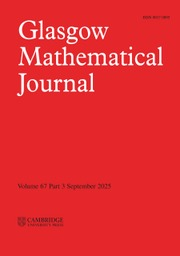Home
Hostname: page-component-5c569c448b-bmzkg Total loading time: 0.225 Render date: 2022-07-02T22:00:35.645Z Has data issue: true Feature Flags: { "shouldUseShareProductTool": true, "shouldUseHypothesis": true, "isUnsiloEnabled": true, "useRatesEcommerce": false, "useNewApi": true } hasContentIssue trueGlasgow Mathematical Journal

# Square roots of hyponormaloperators

Published online by Cambridge University Press:  01 October 1999

## Abstract

HTML view is not available for this content. However, as you have access to this content, a full PDF is available via the ‘Save PDF’ action button.

An operator $T\in$[Lscr ]$(H)$ is called a square root of a hyponormal operator if $T^2$ is hyponormal. In this paper, we prove the following results: Let $S$ and $T$ be square roots of hyponormal operators.

(1) If $\sigma(T)\cap[-\sigma(T)]=\phi$ or {0}, then $T$ is isoloid (i.e., every isolated point of $\sigma(T)$ is an eigenvalue of $T$).

(2) If $S$ and $T$ commute, then $ST$ is Weyl if and only if $S$ and $T$ are both Weyl.

(3) If $\sigma(T)\cap[-\sigma(T)]=\phi$ or {0}, then Weyl's theorem holds for $T$.

(4) If $\sigma(T)\cap[-\sigma(T)]=\phi$, then $T$ is subscalar. As a corollary, we get that $T$ has a nontrivial invariant subspace if $\sigma(T)$ has non-empty interior. (See .)

Type
Research Article
Information
Glasgow Mathematical Journal , October 1999 , pp. 463 - 470
1999 Glasgow Mathematical Journal TrustYou have Access
3
Cited by

# Save article to Kindle

Note you can select to save to either the @free.kindle.com or @kindle.com variations. ‘@free.kindle.com’ emails are free but can only be saved to your device when it is connected to wi-fi. ‘@kindle.com’ emails can be delivered even when you are not connected to wi-fi, but note that service fees apply.

Find out more about the Kindle Personal Document Service.

Square roots of hyponormal operators
Available formats
×

# Save article to Dropbox

To save this article to your Dropbox account, please select one or more formats and confirm that you agree to abide by our usage policies. If this is the first time you used this feature, you will be asked to authorise Cambridge Core to connect with your Dropbox account. Find out more about saving content to Dropbox.

Square roots of hyponormal operators
Available formats
×

# Save article to Google Drive

To save this article to your Google Drive account, please select one or more formats and confirm that you agree to abide by our usage policies. If this is the first time you used this feature, you will be asked to authorise Cambridge Core to connect with your Google Drive account. Find out more about saving content to Google Drive.

Square roots of hyponormal operators
Available formats
×
×